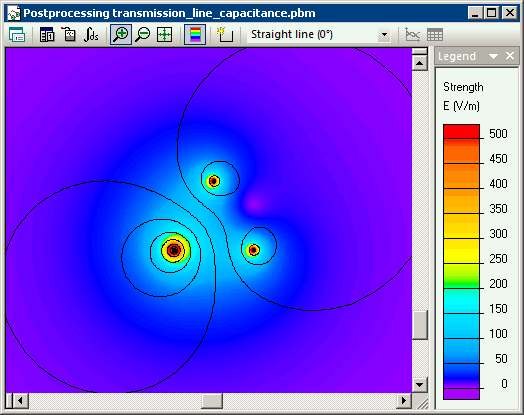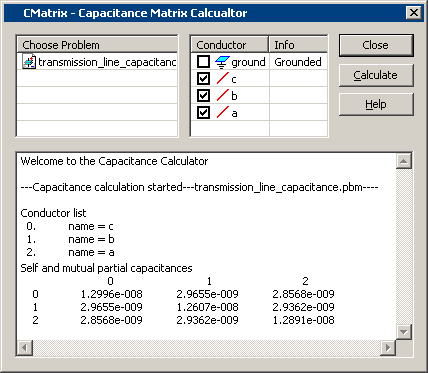Main >> Applications >> Sample problems

# Transmission line capacitance

transmission line capacitance, two wire transmission line electric field, transmission line FEA finite element analysis

3 phase transmission line above earth.

Problem Type
Plane-parallel problem of electrostatics.

Geometryd = 1 m, h = 5 m.
Model depth l = 4 km.

Given
Relative permittivity of air ε = 1,

Solution
Transmission line of 3 conductors features self- and mutual- partial capacitances. It is convenient to use capacitance matrix calculator add-in to find all the partial capacitances.

Results
Electric field distribution around the transmission line.C, nF Phase A Phase B Phase C Phase A 13 3 2.9 Phase B 3 12.6 2.9 Phase C 2.9 2.9 12.9
• Video: Transmission line capacitance
•